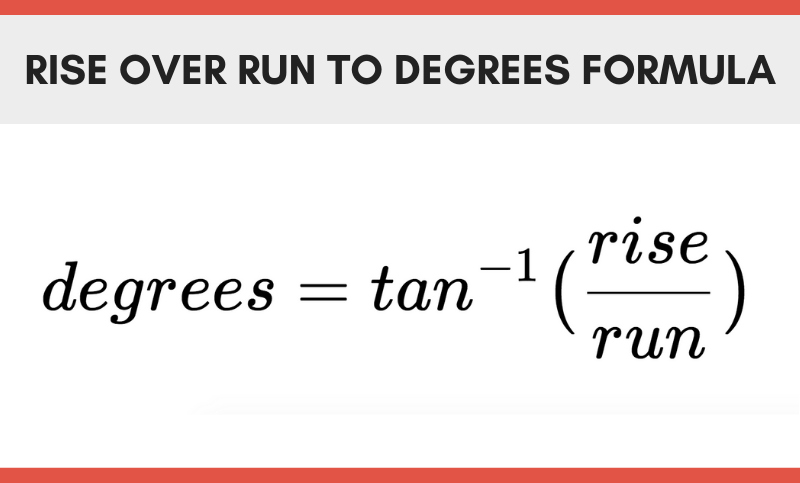# Rise Over Run to Degrees Calculator

Calculate an angle in degrees given the rise and run of an incline. Rise over run can also be referred to as a gradient.

## Angle in Degrees:

Learn how we calculated this below

## How to Calculate an Angle in Degrees using Rise over Run

The formula to calculate the slope of a line is often called rise over run. Rise is equal to the height of a line, and run is equal to the horizontal length of a line.

The rise over run formula for calculating a slope is rise divided by run.

rise / run

We can solve the angle of an incline by finding the rise and the run of a line. First, convert rise and run to the same units of measure.

Then, divide the rise by the run to find the decimal form.

Finally, get the inverse tangent of the decimal to find the angle in degrees.

Thus, the formula to convert rise over run to an angle in degrees is:

degrees = tan-1(rise / run)For example, given a rise of 10′ and a run of 20′:

degrees = tan-1(10 ÷ 20)
degrees = tan-1(.5)
degrees = 26.57°

The slope or incline of a line or elevation can be expressed as a gradient(rise/run), angle in degrees, decimal, or percentage. Our rise and run to percentage calculator can convert a slope to a percentage.

You might also find our grade calculator useful for finding the incline of an elevation.